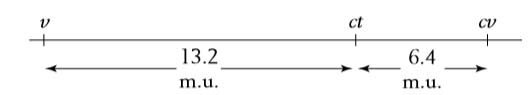# Three-point testcross

is a cross of a trihybrid (triple heterozygote) to a triply recessive tester.

This kind of cross, called a three-point testcross, is a commonly used format in linkage analysis.

The goal is to deduce whether the three genes are linked and, if they are, to deduce their order and the map distances between them.

#### Example

Let’s look at an example, also from Drosophila. In our example,

the mutant alleles are v (vermilion eyes),

cv (crossveinless, or absence of a crossvein on the wing),

and ct (cut, or snipped, wing edges).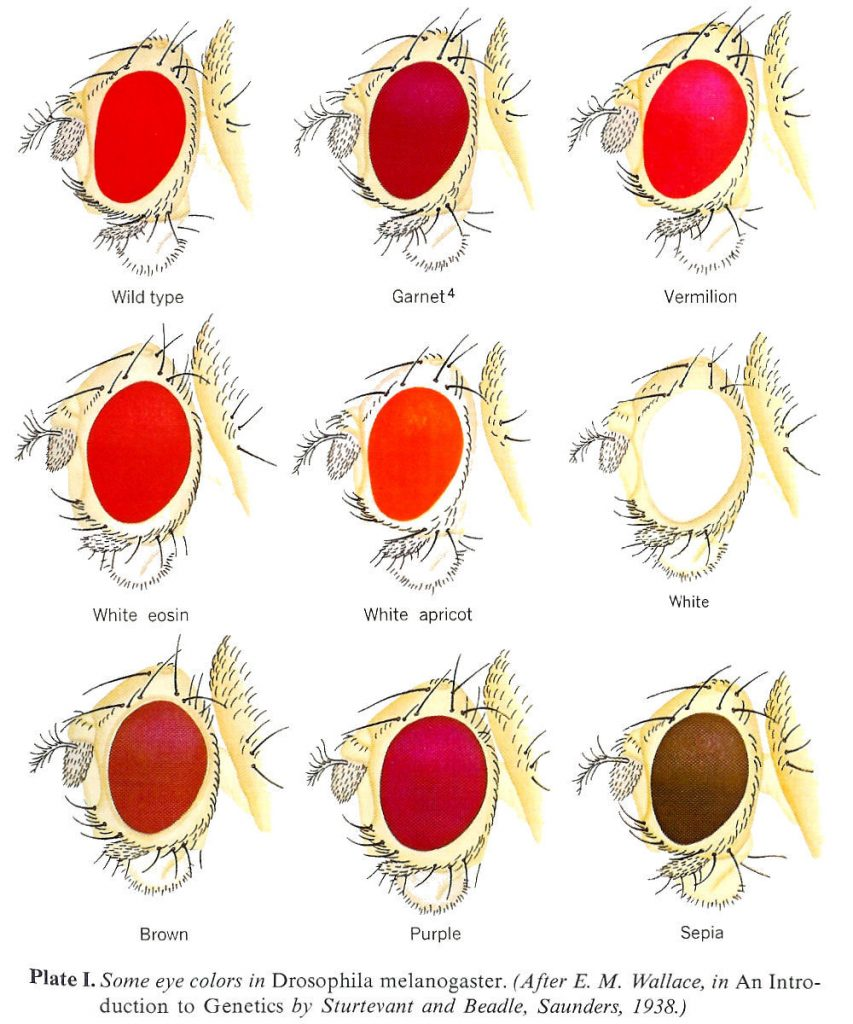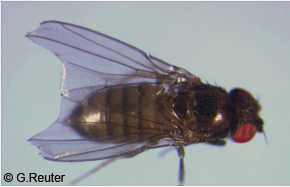fig: cut wing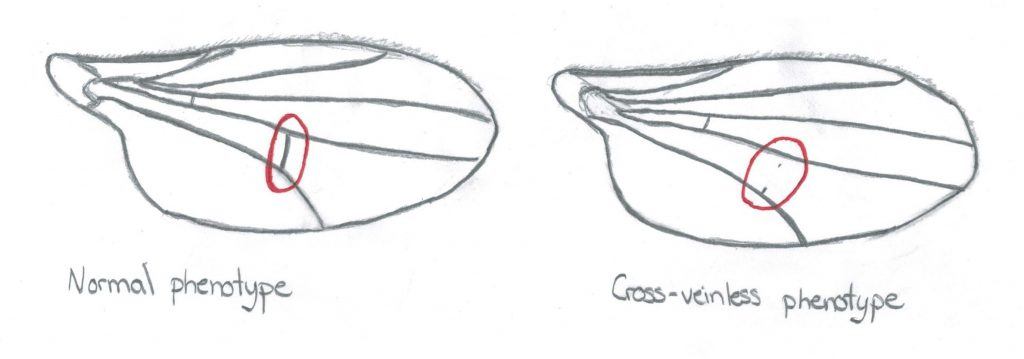Fig: Crossveinless condition in drosophilla a recessive condition

The analysis is carried out by performing the following crosses: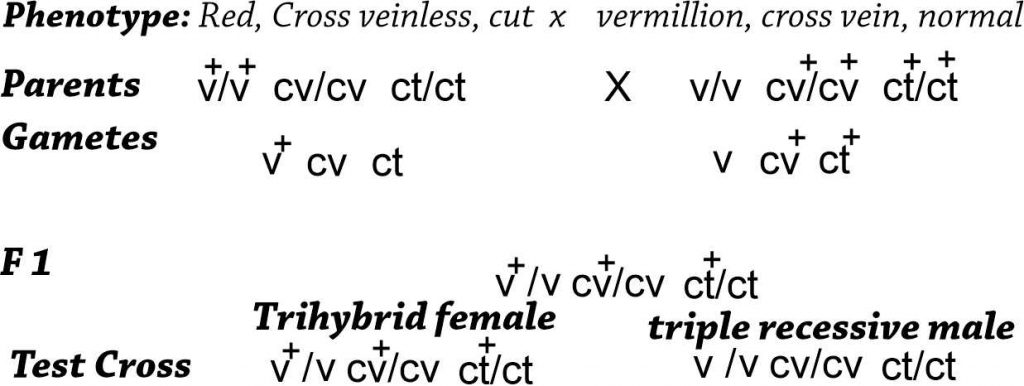From any trihybrid, only 2 x 2 x 2 = 8 gamete genotypes are possible. These are the genotypes seen in the testcross progeny. The chart (on the following page) shows the number of each of the eight gametic genotypes counted out of a sample of 1448 progeny ﬂies.The columns alongside show which genotypes are recombinant (R) for the loci taken two at a time. We must be careful in our classiﬁcation of parental and recombinant types. Note that the parental input genotypes for the triple heterozygotes are v+. cv . ct and v . cv+ . ct+; any combination other than these two constitutes a recombinant.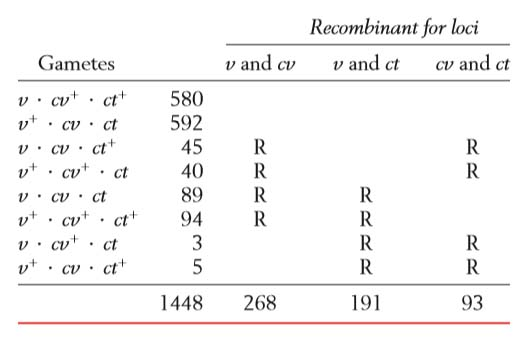#### Recombinations btween c and cv loci

Let’s analyze the loci two at a time,starting with the v and cv loci.

In other words, in the gamete listings we look at just the ﬁrst two columns and cover up the other one. Since the parentals are v . cv+ and v+ . cv,

we know that the recombinants are by deﬁnition v. cv and v+ . cv+.

There are 45 + 40 + 89 + 94 = 268 of these recombinants.

RF= 268/1448 x 100 = 18.5%

Of a total of 1448 ﬂies, this number gives an RF of 18.5 percent.

#### Recombinations btween v and ct loci

For the v and ct loci, the recombinants are v . ct and v+ . ct+ because the parental combinations were v+ . ct and v . ct+

There are 89 +94 + 3 + 5 = 191 of these recombinants among 1448 ﬂies, so the RF = 13.2 percent.

RF = 191/1448 x 100 = 13.2%

#### Recombinations btween cv and ct loci

For ct and cv, the recombinants are cv . ct+ and cv+ . ct.

Parental = cv . ct and cv+ . ct+

There are 45 + 40 + 3 + 5 =  93 of these recombinants among the 1448,

so the RF =  93/ 1448 x 100 = 6.4 %.

because the RF values are all considerably less than 50 percent. Because the v and cv loci show the largest RF value, they must be farthest apart; therefore, the ct locus must lie between them. A map can be drawn as follows: